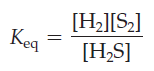×
Get Full Access to Introductory Chemistry - 5 Edition - Chapter 15 - Problem 47p
Get Full Access to Introductory Chemistry - 5 Edition - Chapter 15 - Problem 47p

×

Consider the reaction. Find the mistakes in the equilibrium expression and fix themISBN: 9780321910295 34

Solution for problem 47P Chapter 15

Introductory Chemistry | 5th Edition

• Textbook Solutions
• 2901 Step-by-step solutions solved by professors and subject experts
• Get 24/7 help from StudySoup virtual teaching assistantsIntroductory Chemistry | 5th Edition

4 5 1 342 Reviews
31
4
Problem 47P

Consider the reaction.Find the mistakes in the equilibrium expression and fix them.Step-by-Step Solution:
Step 1 of 3

Solution:

In the given equilibrium equation , we have to find out the expression for Keq

2H2S(g)2 H2 (g) + S2(g)

The equilibrium expression is ,

[H2]2 [S2]

Keq =  ---------

[H2S]2

Step 2 of 3

Step 3 of 3

ISBN: 9780321910295

Unlock Textbook Solution